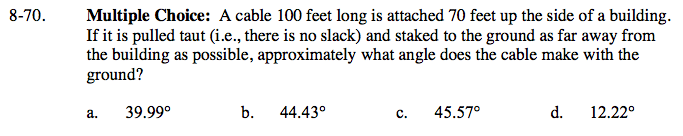Home > GC > Chapter 8 > Lesson 8.2.1 > Problem8-70

8-70.

Multiple Choice: A cable 100 feet long is attached 70 feet up the side of a building. If it is pulled taut (i.e., there is no slack) and staked to the ground as far away from the building as possible, approximately what angle does the cable make with the ground? Homework Help ✎

 a. 39.99° b. 44.43° c. 45.57° d. 12.22°Draw a diagram of this. What inverse trig ratio can you use to find the angle measure?

$\text{(b), because the sin}^{-1}\left(\frac{70}{100}\right) \approx 44.43\degree.$